## Introduction

This tutorial shows step by step process to conduct a bi-variate linear regression analysis for an introductory statistics class.

## Dataset

The “Prestige (available from {car} package in R)” dataset is used in this analysis, which was obtained from Fox, J. and Weisberg, S. (2011) An R Companion to Applied Regression, Second Edition, Sage. The Prestige data set has 102 rows and 6 variables. The observations are occupations.

### Variable Definitons

This data frame contains the following columns:
education: Average education of occupational incumbents, years, in 1971.
income: Average income of incumbents, dollars, in 1971.
women: Percentage of incumbents who are women.
prestige: Pineo-Porter prestige score for occupation, from a social survey conducted in the mid-1960s.
census: Canadian Census occupational code.
type: Type of occupation. bc - Blue Collar; prof - Professional, Managerial, and Technical; wc - White Collar.

#### First 10 rows of the dataset

                    education income women prestige census type
gov.administrators      13.11  12351 11.16     68.8   1113 prof
general.managers        12.26  25879  4.02     69.1   1130 prof
accountants             12.77   9271 15.70     63.4   1171 prof
purchasing.officers     11.42   8865  9.11     56.8   1175 prof
chemists                14.62   8403 11.68     73.5   2111 prof
physicists              15.64  11030  5.13     77.6   2113 prof
biologists              15.09   8258 25.65     72.6   2133 prof
architects              15.44  14163  2.69     78.1   2141 prof
civil.engineers         14.52  11377  1.03     73.1   2143 prof
mining.engineers        14.64  11023  0.94     68.8   2153 prof

### Examine the data before fitting models

   education          income          women           prestige
Min.   : 6.380   Min.   :  611   Min.   : 0.000   Min.   :14.80
1st Qu.: 8.445   1st Qu.: 4106   1st Qu.: 3.592   1st Qu.:35.23
Median :10.540   Median : 5930   Median :13.600   Median :43.60
Mean   :10.738   Mean   : 6798   Mean   :28.979   Mean   :46.83
3rd Qu.:12.648   3rd Qu.: 8187   3rd Qu.:52.203   3rd Qu.:59.27
Max.   :15.970   Max.   :25879   Max.   :97.510   Max.   :87.20
census       type
Min.   :1113   bc  :44
1st Qu.:3120   prof:31
Median :5135   wc  :23
Mean   :5402   NA's: 4
3rd Qu.:8312
Max.   :9517            

Notice that the ‘type’ variable has 4 missing values.

#### Correlation Matrix

           prestige education    income
prestige  1.0000000 0.8501769 0.7149057
education 0.8501769 1.0000000 0.5775802
income    0.7149057 0.5775802 1.0000000

Let’s focus on three variables of our interest - ‘prestige’, ‘education’, and ‘income’. While we observe that prestige is positively correlated with both education and income, education appears to be correlated more strongly than income with prestige.

#### Plot the data before fitting models

Plot the data to look for outliers, non-linear relationships etc.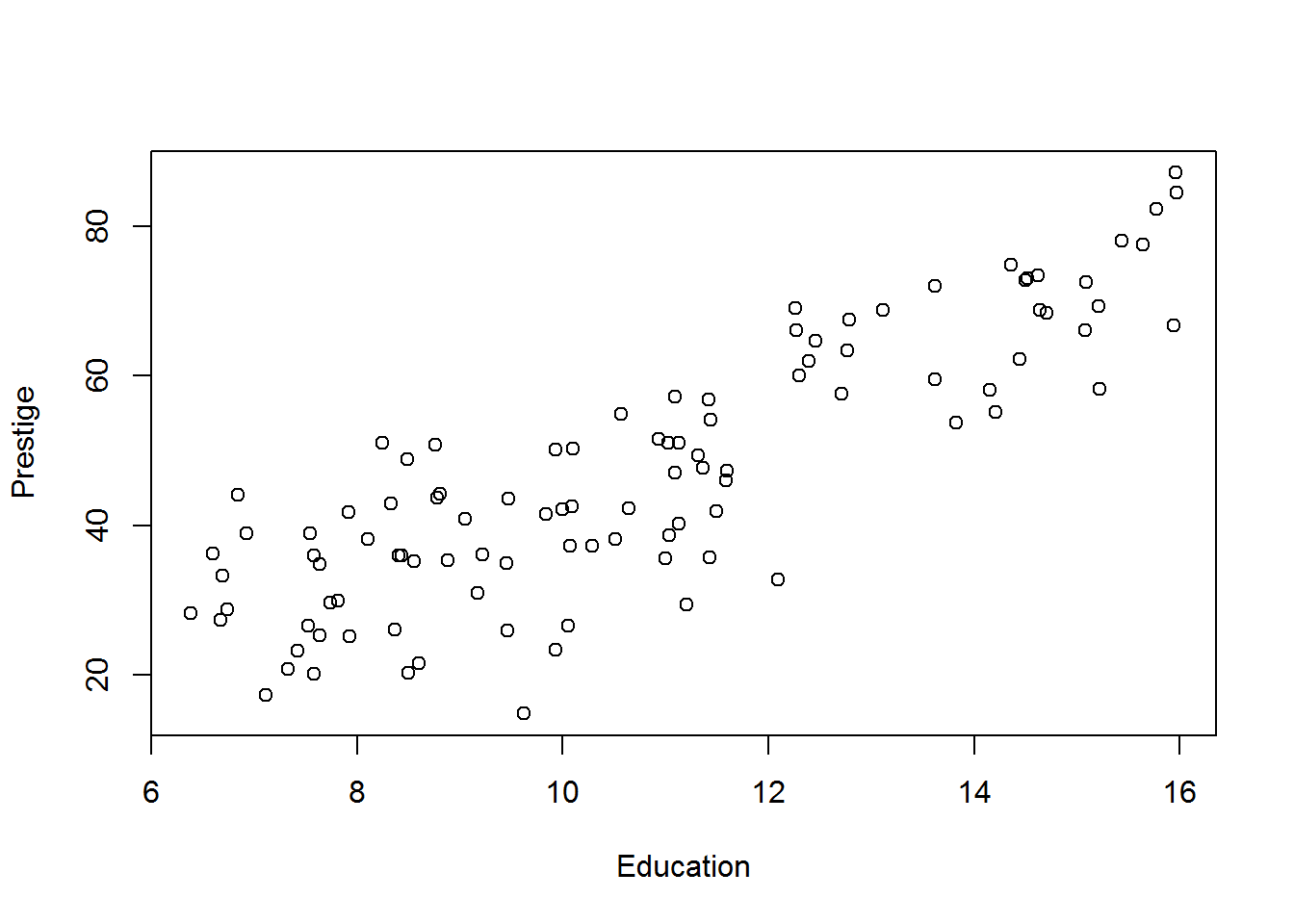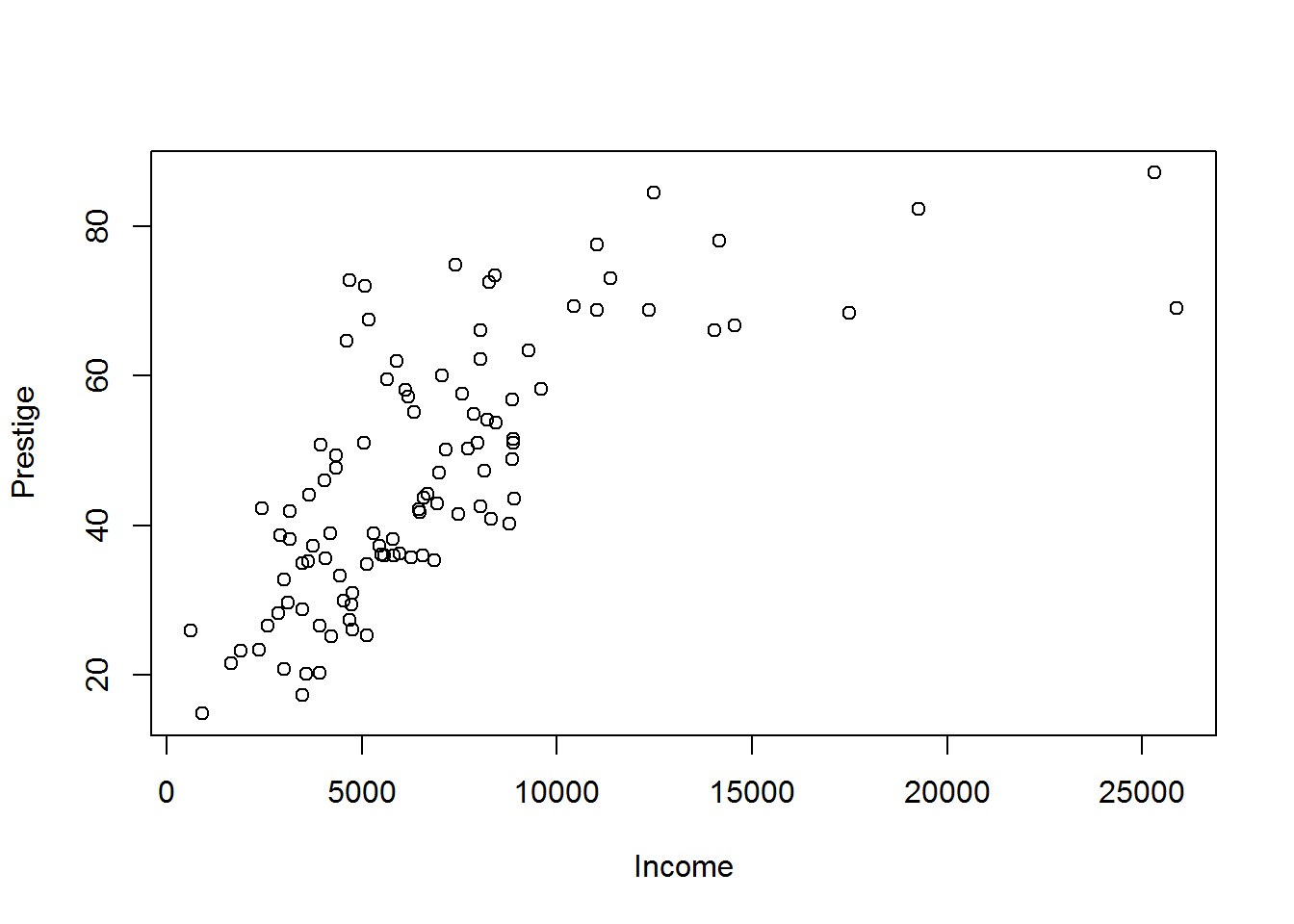As expected, the relationship between education and prestige appears to be more linear than that between income and prestige.

## Linear Regression Model

Next, we fit response variable ‘prestige’ with explanatory variables ‘education’ and ‘income’ separately and find out which variable is the stronger predictor.

### Education Model

$$prestige = b_{0} + b_{1} * education + e$$


Call:
lm(formula = prestige ~ education, data = Prestige)

Residuals:
Min       1Q   Median       3Q      Max
-26.0397  -6.5228   0.6611   6.7430  18.1636

Coefficients:
Estimate Std. Error t value Pr(>|t|)
(Intercept)  -10.732      3.677  -2.919  0.00434 **
education      5.361      0.332  16.148  < 2e-16 ***
---
Signif. codes:  0 '***' 0.001 '**' 0.01 '*' 0.05 '.' 0.1 ' ' 1

Residual standard error: 9.103 on 100 degrees of freedom
Multiple R-squared:  0.7228,    Adjusted R-squared:   0.72
F-statistic: 260.8 on 1 and 100 DF,  p-value: < 2.2e-16

95% confidence interval of the estimated coefficients

                 2.5 %    97.5 %
(Intercept) -18.027220 -3.436744
education     4.702223  6.019533

The education variable appears to be highly significant. The $$R^{2}$$ suggests that the model explains 72% of the variability of the response variable ‘prestige’.

### Residual Plot: Linear Regression Assumptions

• Ordinary least squares regression relies on several assumptions, including that the residuals are normally distributed and homoscedastic, the errors are independent and the relationships are linear.
• Investigate these assumptions visually by plotting the model: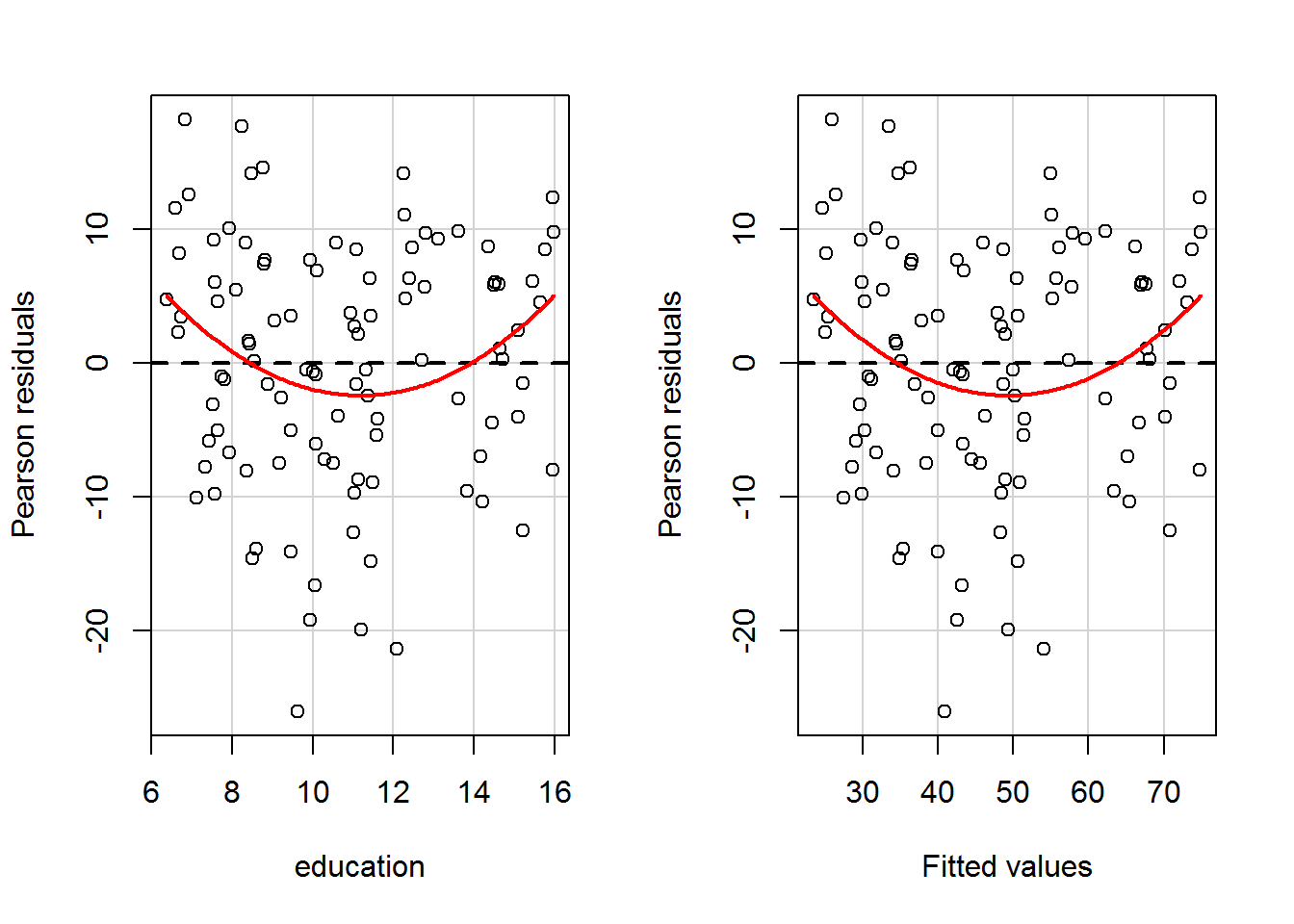Test stat Pr(>|t|)
education      2.596    0.011
Tukey test     2.596    0.009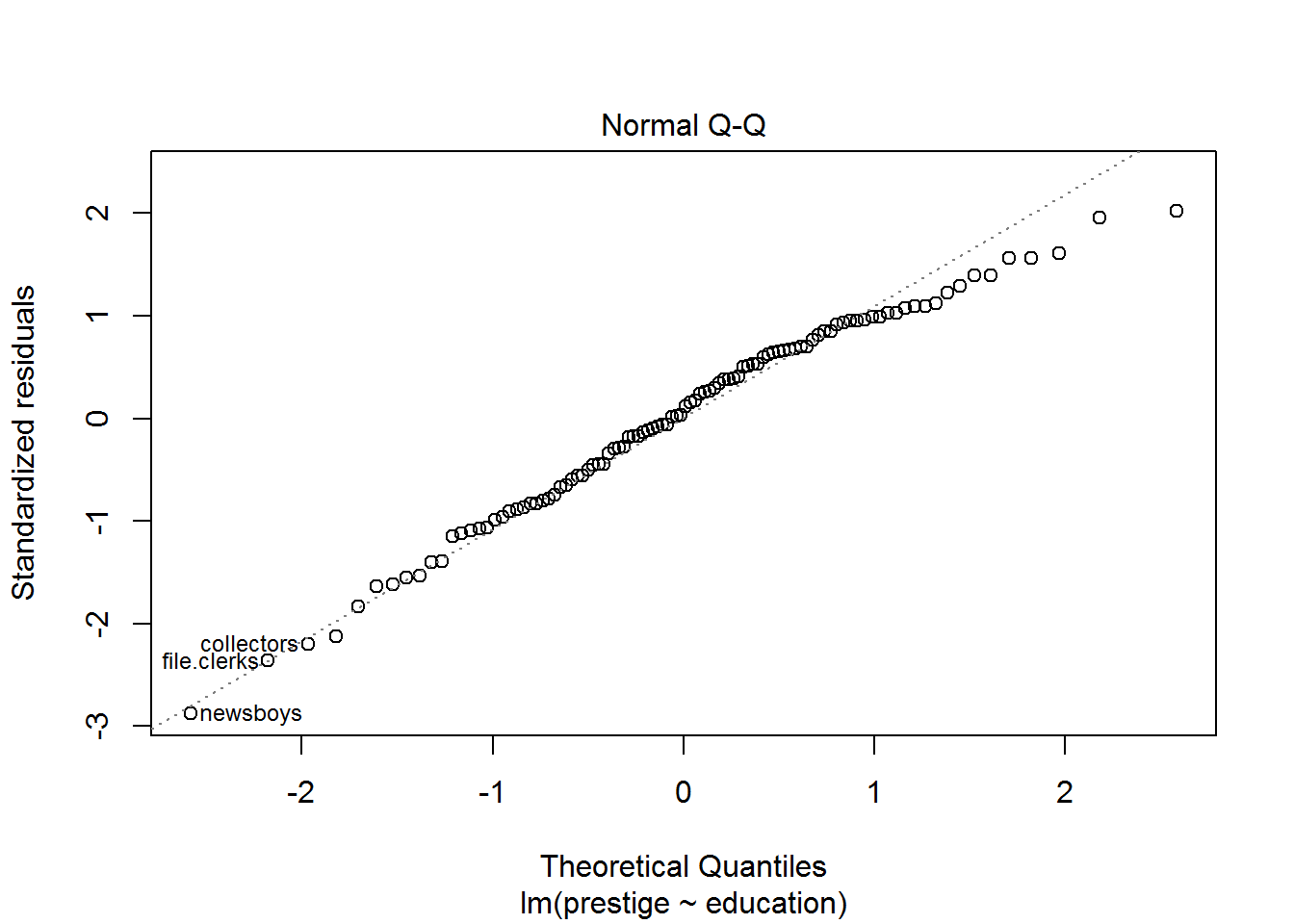We observe that there is no linear relationship between the fitted values of ‘prestige’ and the residuals. Also, the Q-Q plot suggests that the residual is normally distributed (approximately).

### Income Model

$$prestige = b_{0} + b_{1}*log(income) + e$$


Call:
lm(formula = prestige ~ log(income), data = Prestige)

Residuals:
Min      1Q  Median      3Q     Max
-19.114  -9.342  -1.218   8.101  30.454

Coefficients:
Estimate Std. Error t value Pr(>|t|)
(Intercept) -139.856     16.954  -8.249  6.6e-13 ***
log(income)   21.556      1.953  11.037  < 2e-16 ***
---
Signif. codes:  0 '***' 0.001 '**' 0.01 '*' 0.05 '.' 0.1 ' ' 1

Residual standard error: 11.61 on 100 degrees of freedom
Multiple R-squared:  0.5492,    Adjusted R-squared:  0.5447
F-statistic: 121.8 on 1 and 100 DF,  p-value: < 2.2e-16

95% confidence interval of the estimated coefficients

                 2.5 %     97.5 %
(Intercept) -173.49239 -106.21906
log(income)   17.68139   25.43134

Comparing $$R^{2}$$, we can conclude that education is a better predictor of prestige than income.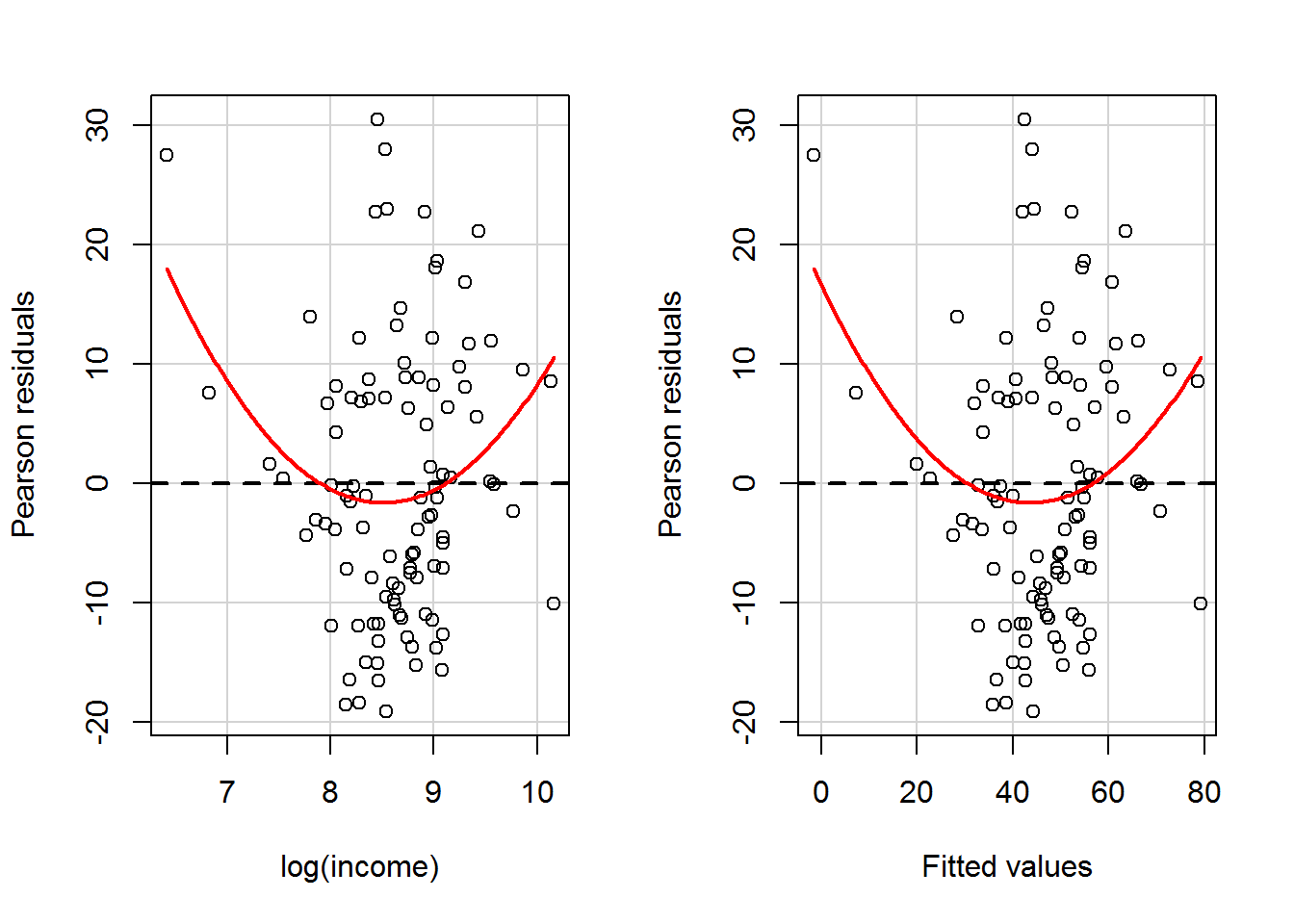Test stat Pr(>|t|)
log(income)     2.694    0.008
Tukey test      2.694    0.007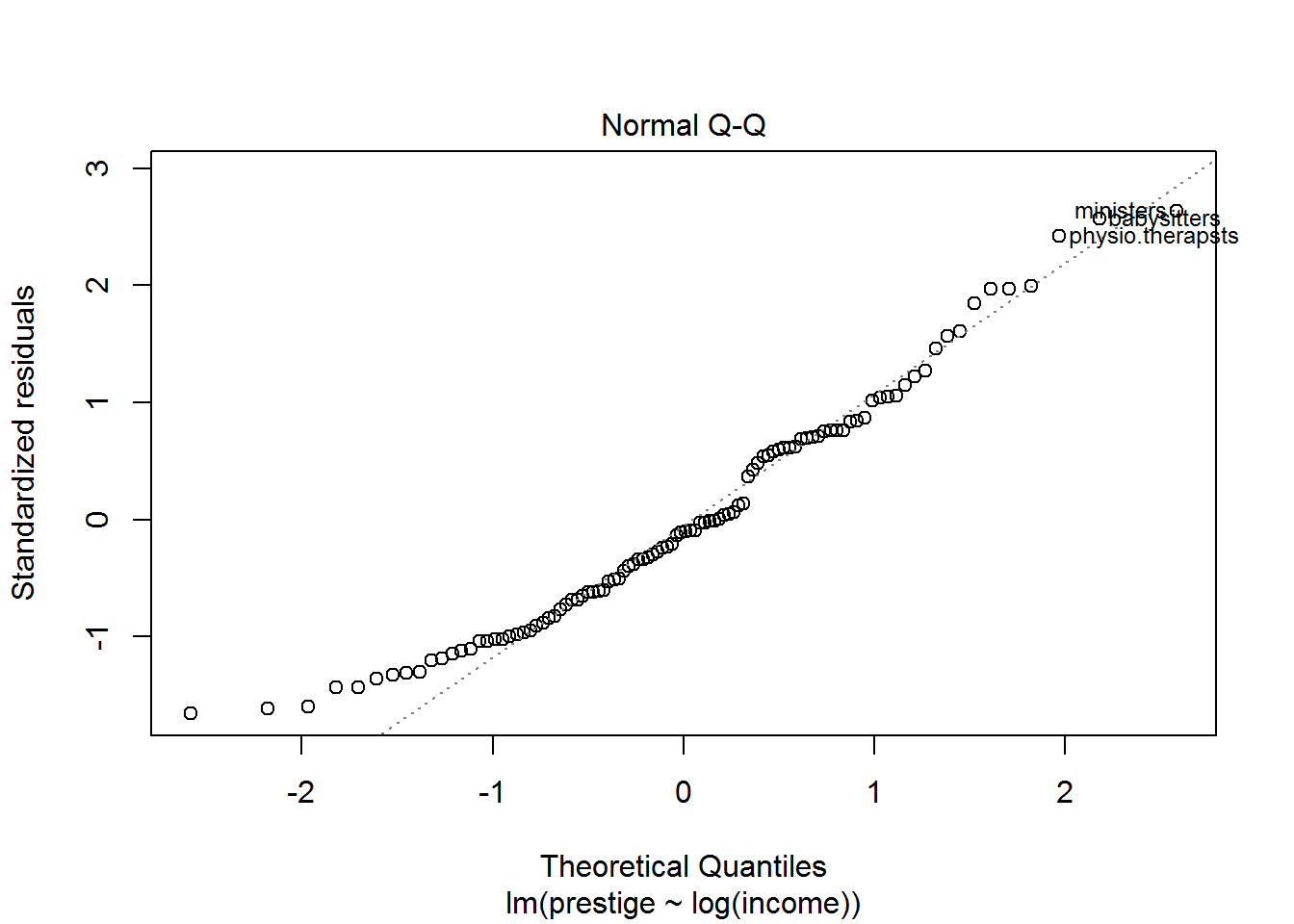## Comparing Models

Do we get a better fit if we use both education and income in the model as predictors of prestige?

### Multivariate Model

$$prestige = b_{0} + b_{1}*education + b_{2}*log(income) + e$$


Call:
lm(formula = prestige ~ education + log(income), data = Prestige)

Residuals:
Min       1Q   Median       3Q      Max
-17.0346  -4.5657  -0.1857   4.0577  18.1270

Coefficients:
Estimate Std. Error t value Pr(>|t|)
(Intercept) -95.1940    10.9979  -8.656 9.27e-14 ***
education     4.0020     0.3115  12.846  < 2e-16 ***
log(income)  11.4375     1.4371   7.959 2.94e-12 ***
---
Signif. codes:  0 '***' 0.001 '**' 0.01 '*' 0.05 '.' 0.1 ' ' 1

Residual standard error: 7.145 on 99 degrees of freedom
Multiple R-squared:  0.831, Adjusted R-squared:  0.8275
F-statistic: 243.3 on 2 and 99 DF,  p-value: < 2.2e-16
                  2.5 %     97.5 %
(Intercept) -117.016298 -73.371676
education      3.383824   4.620081
log(income)    8.585936  14.288979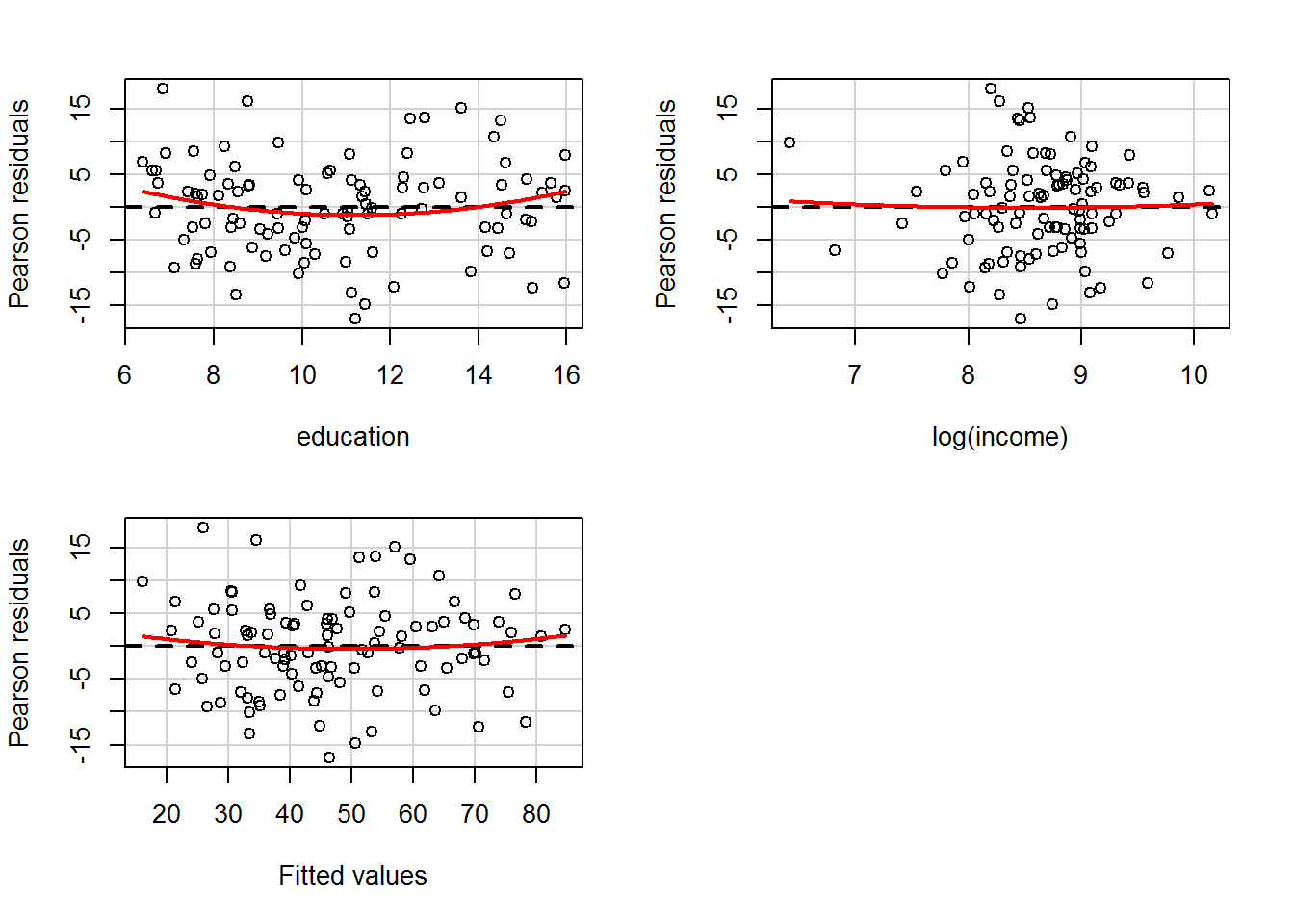Test stat Pr(>|t|)
education       1.615    0.109
log(income)     0.221    0.825
Tukey test      0.653    0.514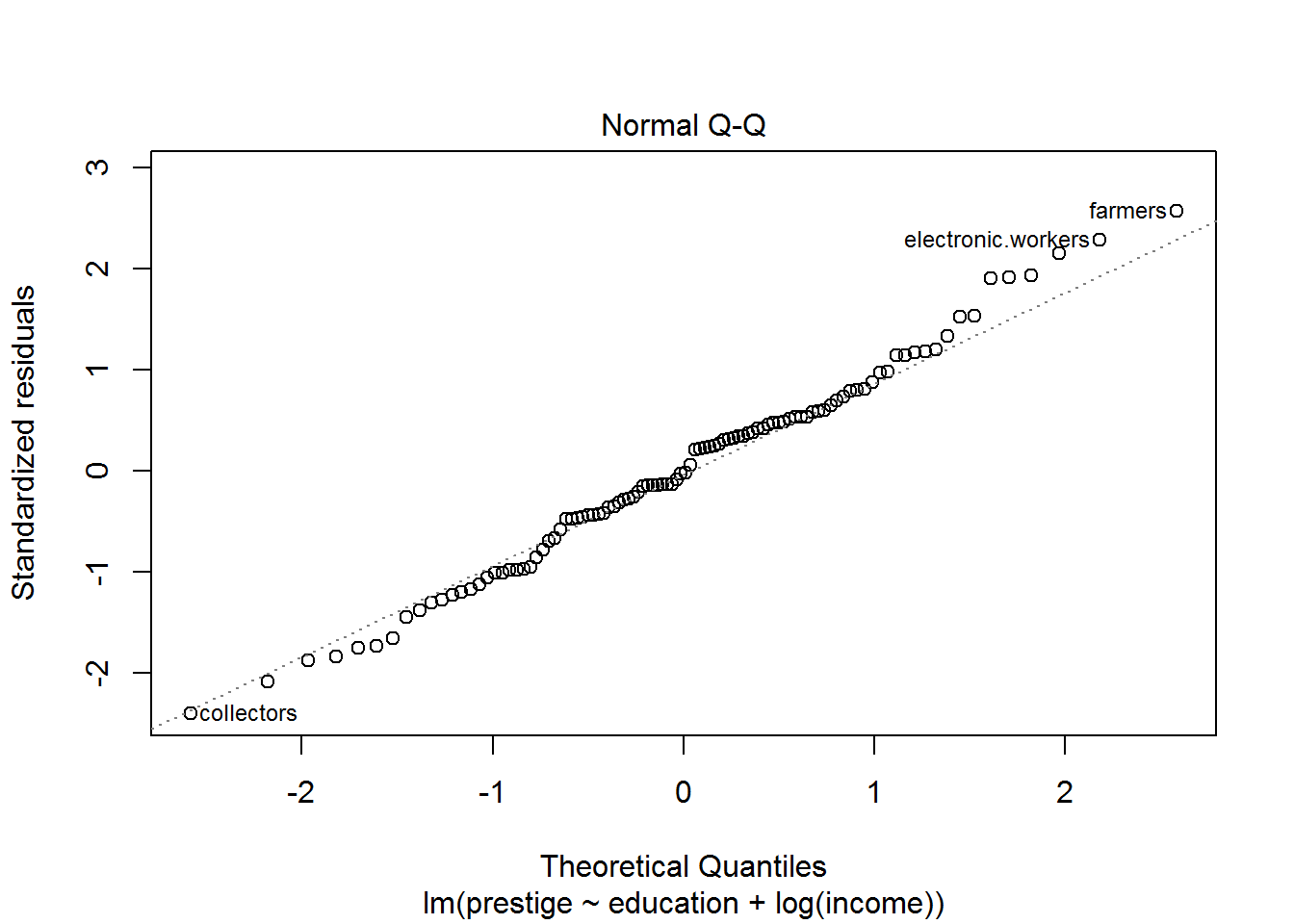#### Compare between education model and multivariate model

Analysis of Variance Table

Model 1: prestige ~ education
Model 2: prestige ~ education + log(income)
Res.Df    RSS Df Sum of Sq      F    Pr(>F)
1    100 8287.0
2     99 5053.6  1    3233.4 63.341 2.942e-12 ***
---
Signif. codes:  0 '***' 0.001 '**' 0.01 '*' 0.05 '.' 0.1 ' ' 1

In this multivariate model, we find that $$R^{2}$$ is further improved. Also, the residual sum of squares is significantly lower than the education and income models. This suggests that combination of education and income is a better predictor of prestige than the bi-variate models.